# 神经网络变得轻松（第二部分）：网络训练和测试

26 十二月 2020, 10:19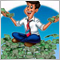5
1 180

### 1. 定义问题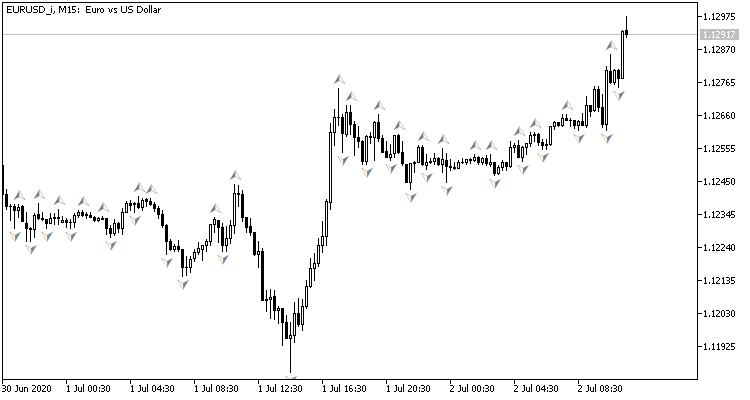• 在正向递进中，我们会将当前行情状况输入到神经网络，并输出最后一根收盘烛条上提取的形成概率的评估。
• 对于逆向递进中，在下一根烛条形成之后，我们将检查前一根烛条上是否存在分形，并将输入结果按权重调整。

### 3. 编程

#### 3.1. 准备工作

• NeuroNet.mqh — 前一篇文章中创建神经网络的函数库
• SymbolInfo.mqh — 接收品种数据的标准库
• TimeSeries.mqh — 处理时间序列的标准库
• Volumes.mqh — 接收交易量数据的标准库
• Oscilators.mqh — 含有振荡器类的标准库

```#include "NeuroNet.mqh"
#include <Indicators\TimeSeries.mqh>
#include <Indicators\Volumes.mqh>
#include <Indicators\Oscilators.mqh>
```

```//+------------------------------------------------------------------+
//|   input parameters                                               |
//+------------------------------------------------------------------+
input int                  StudyPeriod =  10;            //Study period, years
input uint                 HistoryBars =  20;            //Depth of history
ENUM_TIMEFRAMES            TimeFrame   =  PERIOD_CURRENT;
//---
input group                "---- RSI ----"
input int                  RSIPeriod   =  14;            //Period
input ENUM_APPLIED_PRICE   RSIPrice    =  PRICE_CLOSE;   //Applied price
//---
input group                "---- CCI ----"
input int                  CCIPeriod   =  14;            //Period
input ENUM_APPLIED_PRICE   CCIPrice    =  PRICE_TYPICAL; //Applied price
//---
input group                "---- ATR ----"
input int                  ATRPeriod   =  14;            //Period
//---
input group                "---- MACD ----"
input int                  FastPeriod  =  12;            //Fast
input int                  SlowPeriod  =  26;            //Slow
input int                  SignalPeriod=  9;             //Signal
input ENUM_APPLIED_PRICE   MACDPrice   =  PRICE_CLOSE;   //Applied price
```

```CSymbolInfo         *Symb;
CiOpen              *Open;
CiClose             *Close;
CiHigh              *High;
CiLow               *Low;
CiVolumes           *Volumes;
CiTime              *Time;
CNet                *Net;
CArrayDouble        *TempData;
CiRSI               *RSI;
CiCCI               *CCI;
CiATR               *ATR;
CiMACD              *MACD;
//---
double               dError;
double               dUndefine;
double               dForecast;
double               dPrevSignal;
datetime             dtStudied;
bool                 bEventStudy;
```

#### 3.2 初始化类

```//+------------------------------------------------------------------+
//| Expert initialization function                                   |
//+------------------------------------------------------------------+
int OnInit()
{
//---
Symb=new CSymbolInfo();
if(CheckPointer(Symb)==POINTER_INVALID || !Symb.Name(_Symbol))
return INIT_FAILED;
Symb.Refresh();
```

```   Open=new CiOpen();
if(CheckPointer(Open)==POINTER_INVALID || !Open.Create(Symb.Name(),TimeFrame))
return INIT_FAILED;
//---
Close=new CiClose();
if(CheckPointer(Close)==POINTER_INVALID || !Close.Create(Symb.Name(),TimeFrame))
return INIT_FAILED;
//---
High=new CiHigh();
if(CheckPointer(High)==POINTER_INVALID || !High.Create(Symb.Name(),TimeFrame))
return INIT_FAILED;
//---
Low=new CiLow();
if(CheckPointer(Low)==POINTER_INVALID || !Low.Create(Symb.Name(),TimeFrame))
return INIT_FAILED;
//---
Volumes=new CiVolumes();
if(CheckPointer(Volumes)==POINTER_INVALID || !Volumes.Create(Symb.Name(),TimeFrame,VOLUME_TICK))
return INIT_FAILED;
//---
Time=new CiTime();
if(CheckPointer(Time)==POINTER_INVALID || !Time.Create(Symb.Name(),TimeFrame))
return INIT_FAILED;

```

```   RSI=new CiRSI();
if(CheckPointer(RSI)==POINTER_INVALID || !RSI.Create(Symb.Name(),TimeFrame,RSIPeriod,RSIPrice))
return INIT_FAILED;
//---
CCI=new CiCCI();
if(CheckPointer(CCI)==POINTER_INVALID || !CCI.Create(Symb.Name(),TimeFrame,CCIPeriod,CCIPrice))
return INIT_FAILED;
//---
ATR=new CiATR();
if(CheckPointer(ATR)==POINTER_INVALID || !ATR.Create(Symb.Name(),TimeFrame,ATRPeriod))
return INIT_FAILED;
//---
MACD=new CiMACD();
if(CheckPointer(MACD)==POINTER_INVALID || !MACD.Create(Symb.Name(),TimeFrame,FastPeriod,SlowPeriod,SignalPeriod,MACDPrice))
return INIT_FAILED;
```

```#define FileName        Symb.Name()+"_"+EnumToString((ENUM_TIMEFRAMES)Period())+"_"+IntegerToString(HistoryBars,3)+"fr_ea"
...
...
...
...
Net=new CNet(NULL);
ResetLastError();
{
printf("%s - %d -> Error of read %s prev Net %d",__FUNCTION__,__LINE__,FileName+".nnw",GetLastError());

```

```      CArrayInt *Topology=new CArrayInt();
if(CheckPointer(Topology)==POINTER_INVALID)
return INIT_FAILED;

```

```      if(!Topology.Add(HistoryBars*12))
return INIT_FAILED;

```

```      int n=1000;
bool result=true;
for(int i=0;(i<4 && result);i++)
{
n=(int)MathMax(n*0.3,20);
}
if(!result)
{
delete Topology;
return INIT_FAILED;
}

```

```      if(!Topology.Add(1))
return INIT_FAILED;

```

```      delete Net;
Net=new CNet(Topology);
delete Topology;
if(CheckPointer(Net)==POINTER_INVALID)
return INIT_FAILED;

```

• dError - 标准偏差（误差）
• dUndefine - 未定义分形的百分比
• dForecast - 正确预测分形的百分比
• dtStudied — 最后一根已训练烛条的日期。

```      dError=-1;
dUndefine=0;
dForecast=0;
dtStudied=0;
}
```

```   TempData=new CArrayDouble();
if(CheckPointer(TempData)==POINTER_INVALID)
return INIT_FAILED;
//---
bEventStudy=EventChartCustom(ChartID(),1,(long)MathMax(0,MathMin(iTime(Symb.Name(),PERIOD_CURRENT,(int)(100*Net.recentAverageSmoothingFactor*(dForecast>=70 ? 1 : 10))),dtStudied)),0,"Init");
//---
return(INIT_SUCCEEDED);
}
//+------------------------------------------------------------------+
//| ChartEvent function                                              |
//+------------------------------------------------------------------+
void OnChartEvent(const int id,
const long &lparam,
const double &dparam,
const string &sparam)
{
//---
if(id==1001)
{
Train(lparam);
bEventStudy=false;
OnTick();
}
}
```

```//+------------------------------------------------------------------+
//| Expert deinitialization function                                 |
//+------------------------------------------------------------------+
void OnDeinit(const int reason)
{
//---
if(CheckPointer(Symb)!=POINTER_INVALID)
delete Symb;
//---
if(CheckPointer(Open)!=POINTER_INVALID)
delete Open;
//---
if(CheckPointer(Close)!=POINTER_INVALID)
delete Close;
//---
if(CheckPointer(High)!=POINTER_INVALID)
delete High;
//---
if(CheckPointer(Low)!=POINTER_INVALID)
delete Low;
//---
if(CheckPointer(Time)!=POINTER_INVALID)
delete Time;
//---
if(CheckPointer(Volumes)!=POINTER_INVALID)
delete Volumes;
//---
if(CheckPointer(RSI)!=POINTER_INVALID)
delete RSI;
//---
if(CheckPointer(CCI)!=POINTER_INVALID)
delete CCI;
//---
if(CheckPointer(ATR)!=POINTER_INVALID)
delete ATR;
//---
if(CheckPointer(MACD)!=POINTER_INVALID)
delete MACD;
//---
if(CheckPointer(Net)!=POINTER_INVALID)
delete Net;
if(CheckPointer(TempData)!=POINTER_INVALID)
delete TempData;
}
```

#### 3.3. 训练神经网络

`void Train(datetime StartTrainBar=0)`

• count - 学习期计数
• prev_un - 前一学习期内未识别分形的百分比
• prev_for - 前一学习期内分形正确“预测”的百分比
• prev_er - 前一个学习期的误差
• bar_time - 重新计算酒吧日期
• stop - 用于跟踪强制程序终止调用的标志。

```   int count=0;
double prev_up=-1;
double prev_for=-1;
double prev_er=-1;
datetime bar_time=0;
bool stop=IsStopped();
MqlDateTime sTime;

```

```   MqlDateTime start_time;
TimeCurrent(start_time);
start_time.year-=StudyPeriod;
if(start_time.year<=0)
start_time.year=1900;
datetime st_time=StructToTime(start_time);
dtStudied=MathMax(StartTrainBar,st_time);
```

```   do
{
int bars=(int)MathMin(Bars(Symb.Name(),TimeFrame,dtStudied,TimeCurrent())+HistoryBars,Bars(Symb.Name(),TimeFrame));
prev_un=dUndefine;
prev_for=dForecast;
prev_er=dError;
ENUM_SIGNAL bar=Undefine;
```

```      if(!Open.BufferResize(bars) || !Close.BufferResize(bars) || !High.BufferResize(bars) || !Low.BufferResize(bars) || !Time.BufferResize(bars) ||
!RSI.BufferResize(bars) || !CCI.BufferResize(bars) || !ATR.BufferResize(bars) || !MACD.BufferResize(bars) || !Volumes.BufferResize(bars))
break;
Open.Refresh(OBJ_ALL_PERIODS);
Close.Refresh(OBJ_ALL_PERIODS);
High.Refresh(OBJ_ALL_PERIODS);
Low.Refresh(OBJ_ALL_PERIODS);
Volumes.Refresh(OBJ_ALL_PERIODS);
Time.Refresh(OBJ_ALL_PERIODS);
RSI.Refresh(OBJ_ALL_PERIODS);
CCI.Refresh(OBJ_ALL_PERIODS);
ATR.Refresh(OBJ_ALL_PERIODS);
MACD.Refresh(OBJ_ALL_PERIODS);
```

```      stop=IsStopped();

```

```      for(int i=(int)(bars-MathMax(HistoryBars,0)-1); i>=0 && !stop; i--)
{
if(i==0)
string s=StringFormat("Study -> Era %d -> %.2f -> Undefine %.2f%% foracast %.2f%%\n %d of %d -> %.2f%% \nError %.2f\n%s -> %.2f",count,dError,dUndefine,dForecast,bars-i+1,bars,(double)(bars-i+1.0)/bars*100,Net.getRecentAverageError(),EnumToString(DoubleToSignal(dPrevSignal)),dPrevSignal);
Comment(s);

```

```         if(i<(int)(bars-MathMax(HistoryBars,0)-1) && i>1 && Time.GetData(i)>dtStudied && dPrevSignal!=-2)
{
TempData.Clear();
bool sell=(High.GetData(i+2)<High.GetData(i+1) && High.GetData(i)<High.GetData(i+1));
Net.backProp(TempData);
if(DoubleToSignal(dPrevSignal)!=Undefine)
{
if(DoubleToSignal(dPrevSignal)==DoubleToSignal(TempData.At(0)))
dForecast+=(100-dForecast)/Net.recentAverageSmoothingFactor;
else
dForecast-=dForecast/Net.recentAverageSmoothingFactor;
dUndefine-=dUndefine/Net.recentAverageSmoothingFactor;
}
else
{
dUndefine+=(100-dUndefine)/Net.recentAverageSmoothingFactor;
}
}
```

```         TempData.Clear();
int r=i+(int)HistoryBars;
if(r>bars)
continue;
//---
for(int b=0; b<(int)HistoryBars; b++)
{
int bar_t=r+b;
double open=Open.GetData(bar_t);
TimeToStruct(Time.GetData(bar_t),sTime);
break;
}
if(TempData.Total()<(int)HistoryBars*12)
break;
```

```         Net.feedForward(TempData);
Net.getResults(TempData);
dPrevSignal=TempData;
```

```         bar_time=Time.GetData(i);
if(i<200)
{
if(DoubleToSignal(dPrevSignal)==Undefine)
DeleteObject(bar_time);
else
DrawObject(bar_time,dPrevSignal,High.GetData(i),Low.GetData(i));
}

```

```         stop=IsStopped();
}
```

```      if(add_loop)
count++;
if(!stop)
{
dError=Net.getRecentAverageError();
{
Net.Save(FileName+".nnw",dError,dUndefine,dForecast,dtStudied,false);
printf("Era %d -> error %.2f %% forecast %.2f",count,dError,dForecast);
}
}
```

```     }
while((!(DoubleToSignal(dPrevSignal)!=Undefine || dForecast>70) || !(dError<0.1 && MathAbs(dError-prev_er)<0.01 && MathAbs(dUndefine-prev_up)<0.1 && MathAbs(dForecast-prev_for)<0.1)) && !stop);

```

```   if(count>0)
{
dtStudied=bar_time;
}
}

```

#### 3.4. 改进梯度计算方法

```void CNeuron::calcOutputGradients(double targetVals)
{
double delta=(targetVals>1 ? 1 : targetVals<-1 ? -1 : targetVals)-outputVal;
}
```

### 4. 测试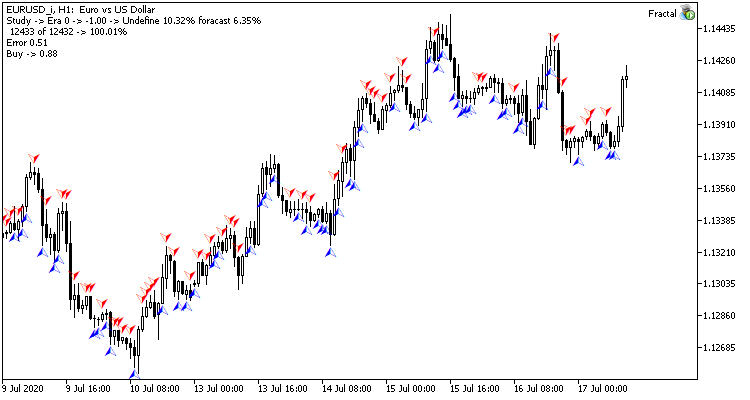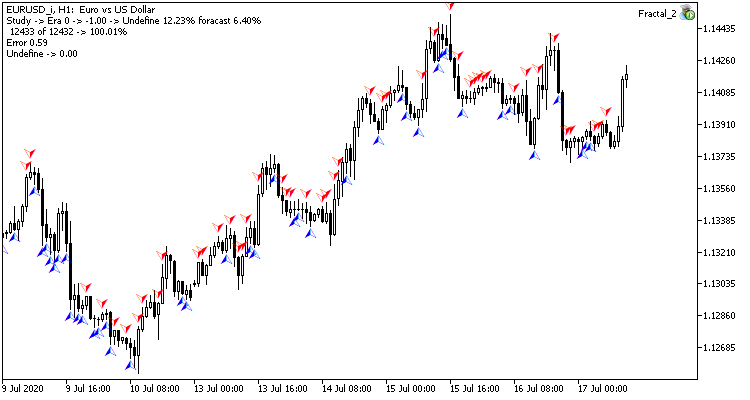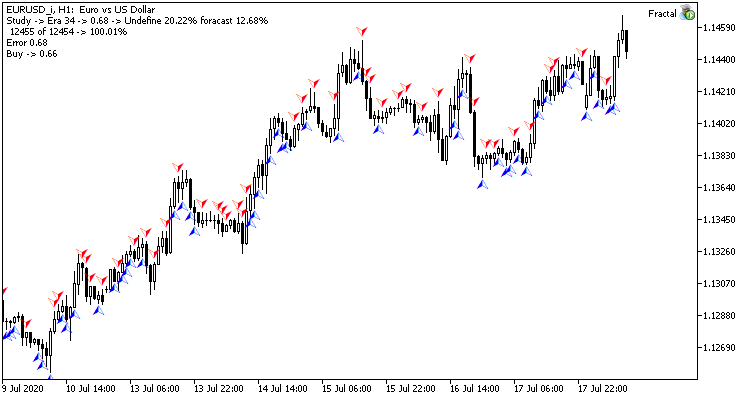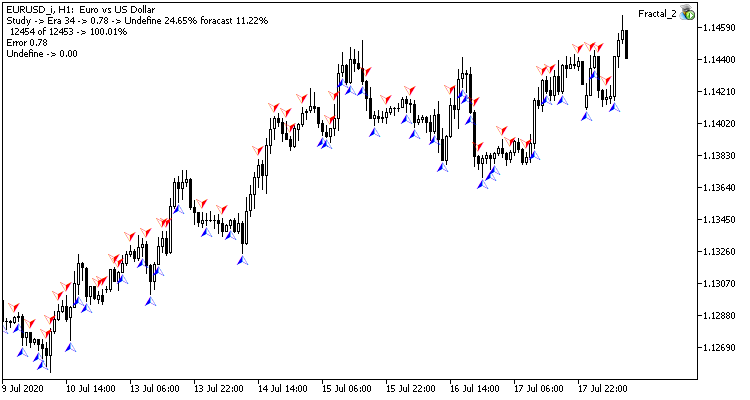### 本文中用到的程序

# 发行 类型 说明
Experts\NeuroNet_DNG\
1 Fractal.mq5   智能交易系统  一款含有回归神经网络（输出层中有 1 个神经元）的智能交易系统
2 Fractal_2.mq5  智能交易系统  一款含有分类神经网络的智能交易系统（输出层中有 3 个神经元）
3 NeuroNet.mqh 类库 创建神经网络（感知器）的类库
Files\
4  Fractal  目录  包含显示回归神经网络测试的屏幕截图
Fractal_2  目录  包含显示分类神经网络测试的屏幕截图

MQL5.zip (2005.76 KB)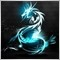| 26 12月 2020 在 10:54
12.68%的命中率？有实际意义吗？我投硬币也50%左右的概率了。这么低的概率，不知道这个东西有何用？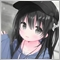| 6 1月 2021 在 21:54
Zhiqiang Zhu:
12.68%的命中率？有实际意义吗？我投硬币也50%左右的概率了。这么低的概率，不知道这个东西有何用？| 6 1月 2021 在 22:00
Zhiqiang Zhu:
12.68%的命中率？有实际意义吗？我投硬币也50%左右的概率了。这么低的概率，不知道这个东西有何用？| 9 1月 2021 在 17:50
Shi Chao Ma:| 9 1月 2021 在 17:51
Shi Chao Ma: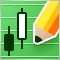自定义品种（符号）：实践基础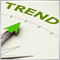什么是趋势，行情结构是基于趋势还是横盘？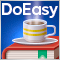DoEasy 函数库中的时间序列（第四十八部分）：在单一子窗口里基于一个缓冲区的多周期、多品种指标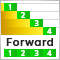连续前行优化 (第八部分): 程序改进和修复